## Introduction

The chemical reactions and gene regulatory networks in living organisms, ecosystems and social communities are all open complex systems. In such systems, complexity emerges, or at least persists, with the successive introductions of new elements. Following the discovery of the general instability of large and complex dynamical systems1 and the development of the theory of the origin of this instability2, the understanding of the stability of such systems has been drastically improved3,4,5,6,7, especially for ecosystems8,9,10,11,12,13,14,15,16,17,18. In an effort to understand large and complex open systems such as ecosystems, various theoretical models that contain evolutionary assembly mechanisms have been proposed6,11,12,13,19,20,21,22,23. In these models, the system typically begins as a small community and new species are gradually introduced. Whether the system can grow by accommodating the newly introduced species or will be disrupted or even destroyed by such a severe disturbance is determined by the various dynamics or rules intrinsic to the system. Interestingly, these models sometimes allow the system to contain a large number of species or high diversity, depending on various parameters of the models.

In addition, simpler models, which do not have dynamical equations of motion and hence do not behave as dynamical systems, have contributed much to the discovery of other general characteristic features of evolving complex systems, such as self-organised criticality5 and the robustness of complex networks24,25,26,27,28,29,30,31. However, these models lack any mechanism to allow the system to grow or shrink depending on the interactions within it. Therefore, an open question remains regarding how and when, in general, such open systems can evolve towards complex structures under successive additions. The purpose of this study is to gain a universal and simple understanding of the basic condition required for a system to grow with the successive introductions of new species. For this purpose, we consider the simple process described below, which was originally proposed to model ecosystems on an evolutionary time scale32.

## Results

### The model

In the present model, the entire system is structured as a collection of nodes connected by directed links with weights (Fig. 1). The nodes may represent chemicals, genes, animals, individuals, or other species; for generality, we simply refer to “species” in the following discussion. In addition, the links may represent many diverse types of interactions among them. The influence of species j on species i is denoted by the weight of the link from node j to node i, aij. Each species has only one property, “fitness”, which is solely determined by the sum of its incoming interactions from other species in the system: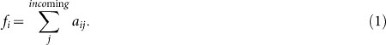Each species can survive as long as its fitness is greater than zero; otherwise, it goes extinct. We calculate the fitness for each species and identify the species with minimum fitness. If the minimum fitness is non-positive, we delete this species. Because this extinction will modify the fitness of the other species, we re-calculate the fitness and re-identify the least-fit species. We continue this deletion procedure until the minimum fitness is positive, meaning that the system is stable.

After finding a stable state, we proceed to the next time step. In each time step, a new species is added into the system. We establish m interactions from/to the new species. The interacting species are chosen randomly from among the resident species with equal probability 1/N(t) and the directions are also determined randomly (the probability to select each of the two directions is 0.5). The link weights are again assigned randomly using the standard normal distribution. Then, we re-calculate the fitness of each species to find the species that should become extinct. When the system returns to a stable state, we again proceed to the next time step for another introduction event. For the initial condition, we begin from a system that consists of N0 species randomly connected with M0 interactions, typically with N0 = 100 and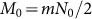. However, it is worth noting that the initial condition is not relevant to the behaviour of the system after a sufficiently large number of time steps (see An incubation rule in Methods). Therefore, our model has only one relevant parameter: the number of interactions per species, m.

### Transitions in growth behaviour

According to this model, can the system grow to become a complex structure? The answer is both yes and no. Although the number of species N(t) sometimes increases and sometimes decreases, its long-term trends can be clearly classified into two cases: either the system grows infinitely (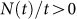, diverging phase), or it remains within a finite range and occasionally dies out (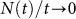, finite phase). The diverging phase appears only for moderate numbers of interactions, 5 ≤ m ≤ 18, while too many or too few links yield the finite phase (Fig. 2).

The reason for the transition from the finite phase to the diverging phase between m = 4 and 5 is relatively simple. Because the species are connected by directed links, the probability for a given species to have an incoming link with positive weight is roughly expected to be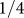. Therefore, in systems with m = 4 or less, each surviving species has an average of only one positive incoming link and the presence of at least one positive incoming link is necessary for survival. This condition means that, although the system sometimes grows large, the structure of the emerging network remains tree- and cycle-like. Such networks are extremely fragile against the removal of certain nodes and therefore they cannot grow with the successive introduction of new nodes. In reality, the probability for a given node to have an incoming link of positive weight is a conditional probability and, hence, can differ from 1/4. Therefore, as we will see below, this transition point may be located below m = 4, for example, instead of between 4 and 5, for a slightly modified model, although the mechanism remains the same.

### The mechanism of the novel transition in robustness

The mechanism of the second transition, between m = 18 and 19, is more complex and fascinating. Because the networks in this regime are not tree-like, this transition is completely unrelated to the mechanism of the previous transition. It also does not stem from certain network structures or motifs. We can confirm that there is no strong structure in the emerging networks. For example, the degree distribution of the system has a peak at m with exponential tails for both sides and the degree-degree correlation is small in the broad range of m ≥ 5 (|assortativity coefficient33| ≤ 0.05) including the critical regime m ~ 18. The clustering coefficient is also confirmed to be small. Therefore, such network is essentially an Erdös-Rényi random graph with an average number of links m. We can also confirm that there are no evident correlations among link weights.

Under the assumption of such a correlation-less structure for the emerging networks, the following process should well approximate the temporal evolution of the system. In our model, every change in the fitness of each species arises from the addition/deletion of an in-coming link. Link addition occurs when a new species is introduced and deletion occurs when a species goes extinct. If we can calculate the average probability E of a resident species going extinct during such a link addition/deletion event, the average number of species that go extinct directly because of the introduction of the new species is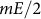(Fig. 3 right). Because these extinctions may also trigger sequential extinctions, the expectation value of the total number of extinctions per addition of one species into a system in which all species have m interactions can be calculated as an infinite geometric series: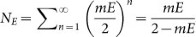. Because NE = 1 means that the number of extinctions is equal to the number of additions in the long-term average, mE = 1 corresponds to the transition point.

The remaining task for the estimation of the critical value of m is to calculate E as a function of m. Because the newly assigned link weight is chosen using the standard normal distribution, the introduction of a new species causes the connecting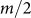species to undergo one step of a symmetric random walk in their fitness. For species deletion events, the change in fitness includes a negative drift that is proportional to the fitness fi. This drift arises simply because the sum of the weights of incoming links, one of which is being lost, yields the current fitness. Therefore, for the fitness distribution, one link addition/deletion event acts as a convolution process. Because a species with negative fitness becomes extinct, the portion of the distribution that falls in the negative fitness range is removed after the convolution (Fig. 3 left). Beginning from the fitness distribution function of newly added species, that is, the positive half of the Gaussian distribution of deviation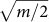, we perform this convolution-and-cut process repeatedly to obtain the fitness distribution of the “elder” generations (in terms of their experience of the link-change events). After finding all distribution functions for different “generations”, we obtain the fitness distribution function of the entire community. Then, the average area ratio of the negative region produced after the convolution is performed on the entire fitness distribution gives the average extinction probability E (see Calculation of the extinction probability per link change in Methods for the detail).

The extinction probability E and the related quantity mE, as numerically calculated from this convolution process, are shown in Fig. 4. As confirmed in this figure, the extinction probability E decreases with increasing m because a larger value of m makes the fitness distribution broader. However, this decrease is slower than m−1. Therefore, mE slowly increases with m and crosses the critical value 1 at approximately m* = 13. Considering the rough approximation we used and the slow increase of mE with m near the critical point, the agreement with the simulation result (m* = 18.5) is rather good.

The mechanism we have identified is valid for slightly different models, as well (Fig. 5). One example is a model in which the number of interactions of each new species is chosen from a uniform distribution in the interval (1, M). The same diversifying transition occurs between M = 35 and 36, i.e., the average number of interactions is approximately 18 ~ 19. Modifying the weight distribution, to a uniform distribution, for example, also results in only a small shift in the transition points (Fig. 5 (a)). Therefore, the global structure of the transition behaviour is universal for these modified models. For a model in which the interaction density ρ is specified, instead of the interaction number m, the transition again occurs at = m*. This means that the number of species fluctuates around a fixed value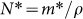(Fig. 5 (b)). Therefore, in this case, the present theory allows us to understand and control the resulting average system size32.

## Discussion

These results confirm that our model and the transition mechanism provide a general understanding of how and to what extent a gradually assembled system becomes robust against the further addition of elements: the average fitness of the surviving elements becomes slightly larger under successive addition, as a result of a weak selection in the fitness. It is also clear why elements with extremely large fitness cannot appear and hence, neither the community nor any particular element can become infinitely robust: the better the fitness, the stronger the negative drift the element feels at the extinction of another species in the same community, solely because the current situation is good for it.

In the classical diversity-stability relation that is known for dynamical systems1,2, an intrinsic stability is provided for each element to ensure that it is stable if it has no interactions. For the system to remain stable, each element may have essentially only one interaction that is comparable to the given intrinsic stability in strength. A main strategy to overcoming this problem has been the introduction of a proper condition into the interaction structure11,12,13,14,15,17,18,19,20. In our model, however, the elements have no intrinsic stability: an element with no interaction immediately goes extinct. Even so, the system may grow even when each element has more than 15 interactions. In this sense, the condition we have identified, using a totally different framework, is looser. The mechanism we have found is also different from the discovery on the robustness of complex network24,25,26,27,28,29,30,31, because the it is unrelated to the complex network structure. It should also be noted that near the growth transition point, our system is not in a critical state, in the sense of SOC models5, although the number of species obeys a neutral random walk, which has sometimes been regarded as a hint of critical behaviour. Although there is a cascading extinction process, which is crucial to the determination of the transition point, the distribution function of the avalanche size is essentially exponential even at the critical point, as it is explained in the theory (Fig. 6). This keeps the resulting system robust against the entirely random incursions. The avalanche size distribution exhibits a fatter tail in the regime below the first transition (m = 4, for instance), which is understood to be an indication of the fragility originating from the tree- or cycle-like structure.

Because the model is simple and abstract, it may be applicable to a broader class of problems, such as social and economic systems. A good example is the characteristic distribution function of the lifetime of elements. Our model, with a fixed interaction density, predicts a stretched exponential function with an exponent of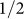for the lifetime distribution32. This result is consistent with the distributions observed in ecosystems (species lifetime distribution in fossil data34,35) and in an economic system (lifetime distribution of the retail goods in Japanese stores36). The basic causes of this characteristic functional form have been found to be an age-insensitive mortality rate and a system-size-independent fluctuation in the number of elements. We can confirm both these properties using the current theory.

## Methods

### An incubation rule

It may be questioned whether the initial condition we chose is sufficiently general and hence whether there could be less restrictive initial conditions that allow the system to grow. To test this possibility, we introduced an incubation rule: totally isolated species (i.e. with a fitness of 0) were allowed to survive when the number of species was below a certain threshold. This procedure prevents total extinction and provides the system with many more opportunities to search for growth from different initial conditions. However, even with this rule implemented, the fact that a system with m in the finite phase remains in a finite range does not change. The average number of species and the average speed of divergence plotted in Fig. 2, the average speed of divergence plotted in Fig. 5 and the long-term series shown in Fig. 7 were obtained using such simulations.

### Calculation of the extinction probability per link change

Because the weight of each newly assigned link is chosen using the standard normal distribution, the introduction of a new species causes the connected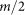species to undergo one step of a symmetric random walk in their fitness. For deletion events, the change in fitness includes a negative drift that is proportional to the fitness fi. This drift arises simply because the sum of the weights of the incoming links, one of which is being lost, yields the fitness. The actual measure of time for each species is the number of link addition/deletion events that the species has experienced, i.e. the number of neighboring species that have been introduced or that has become extinct, not the system time. Therefore, we call this measure the “generation” of the species. The evolution of the (not normalised) distribution function of the fitness under the successive addition of new species with m interactions can be expressed as a convolution process with a cut-off at 0 as follows: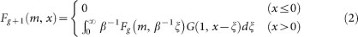where Fg is the fitness distribution function of species of generation g and G (σ, x) is the Gaussian distribution with standard deviation σ. The contraction factor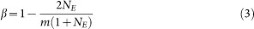represents the strength of the negative drift of the random walk. Because β is a decreasing function of NE and E is an increasing function of β, we can use the value in the neutral regime (NE = 1 and therefore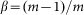) to assess the transition point. The initial condition of the distribution function is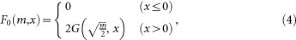as the newly added species has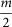incoming links on average and the weight of each incoming link is drawn from the Gaussian distribution G (1, x). The integral of Fg over the entire interval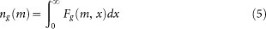gives the probability of a species, once settled in the system, surviving up to generation g (therefore, n0 = 1). From this quantity one can directly calculate the average extinction probability of a species during one link-change event aswhere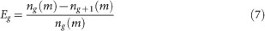is the extinction probability of a species of generation g during an increment in generation.